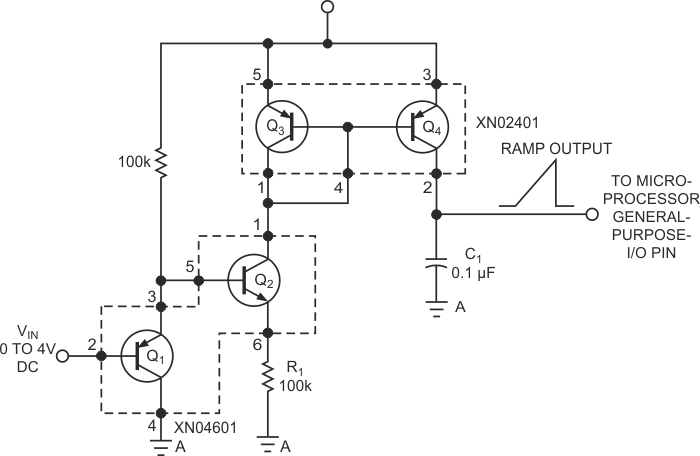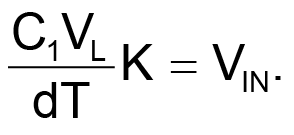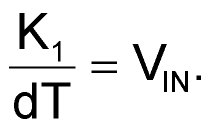# Analog-input circuit serves any microcontroller

## Panasonic XN02401 XN04601

The simple ADC in Figure 1 is perfect for getting analog signals into a purely digital microcontroller. Using just five surface-mount parts, you can assemble it for less than 50 cents (1000), which is approximately half the cost of a single-chip-ADC approach in the same volume. Moreover, this design takes only one pin from the microcontroller to operate. Although you can purchase many microcontrollers with built-in ADCs, in some circumstances, this solution is impractical. For example, you might have an all-digital microcontroller already designed in. In this design, a USB-compatible, digital-only microcontroller needed analog input at low cost for a consumer application. The basic analog portion of the circuit in Figure 1 uses clever transistor arrays from Panasonic. Q1/Q2 and Q3/Q4 are single-package, multiple-transistor arrays. The Q1/Q2 array forms a voltage-to-current converter. The voltage on Q1’s emitter is a diode drop higher than the voltage on Q1’s base. The VBE drop in Q2 returns the original input voltage to the top of R1; R1 then converts that voltage to a current.Figure 1. With two transistor arrays and three discrete components, you can configure an analog front end for a microcontroller.

The Q3/Q4 array forms a standard current-mirror circuit. The current flowing in Q3’s collector matches the current forced in Q4’s collector.Q4’s collector has high impedance, so Q4 provides a suitable current source. The current from Q4 charges C1 at a rate that is proportional to the input voltage. The values in Figure 1 allow for a range of conversion times of 3 msec for an input of 4 V to 56 msec for an input of 0.1 V. The design exploits the fact that most general-purpose microcontrollers have a bidirectional I/O-port structure. That is, you can program a port pin as either an input or an output. When you set a pin as an input, it has very high input impedance, so it can follow the ramp as C1 charges up. When you program a pin as an output, you can set it low, and it discharges C1 for the next conversion cycle. This action gives you the basic operation of a single-slope analog-to-digital-conversion cycle. The basic operations are as follows:

1. Set the ADC pin as a low output to discharge C1.
2. Reset a suitable timer-counter in the microcontroller.
3. Set the ADC pin as an input.
4. Allow the timer to count until it reads as logic 1 in the microcontroller, or let the timer count to some suitably long value, which suggests that the input is essentially zero.
5. Stop the timer counter.
6. Convert to the timer count by some suitable scaling factor to an ADC reading.
7. Start over for the next conversion.

The conversion from the ramp time to a logic 1 on the microcontroller pin depends on the following factors:

• the logic-1 switching level of your microcontroller;
• the input voltage and, hence, the ramp rate of C1;
• the value of C1, which sets the ramp rate;
• the value of R2, which sets the ramp rate; and
• the microcontroller's timer resolution.

You can boil down these variables to the following equation:where VL is the voltage level of the microcontroller's zero-to-one conversion, K is the scaling factor that relates to the voltage-to-current conversion of the input stage and timer resolution, and dT is the time count of the conversion cycle. Because C1VL is also a constant for a given circuit, you can combine it with K to form a single conversion constant of K1. Hence, you can reduce the equation toIn this case, the test code was written for Microchip Technology's PIC16F84 microcontroller. This device has a measured VL of 1.28 V; the counter has a resolution of 1 µsec. It's probably best to empirically determine the factor K1. Set up the counter resolution as desired, allow the microcontroller to make and display that conversion time or send it through a debugger, and, given that you have an exact VIN, K1 is then easy to determine. In this case, K1 turned out to be 2 V×5700 µsec = 11,400.

The constant K1 serves to convert the raw timer count to a voltage. To obtain high resolution, you normally use floating-point math. If you need to display the value, floating-point math might be appropriate, but most applications entail reading a potentiometer or some other system level. In such applications, the output is a bar-chart display or some control value. Thus, you waste microcontroller resources by using floating-point math throughout the conversion process. With careful selection of circuit components, fixed-point math can usually provide, for example, an 8-bit representation (0 to 255) for an input range of 0 to 4 V. If you scale the timer/counter by 64, instead of a count of 5700 µsec for an input of 2 V, you obtain 89. Then, if you want this 89 to correspond to a half-scale value of 128, the value of K1 becomes 11,392. A 16-bit unsigned word easily accommodates this value, and you need no floating-point math in the conversion. The accuracy of this ADC is approximately 5% with no adjustments. The resolution is a function of the timer resolution and how tight the code makes the conversion loop. The resolution can be many times the absolute accuracy. Moreover, the converter is monotonic.

## Materials on the topic

EDNYou may have to register before you can post comments and get full access to forum.
 User Name Remember Me? Password